# Plotting single cell data with schex

Reduced dimension plotting is one of the essential tools for the analysis of single cell data. However, as the number of cells/nuclei in these these plots increases, the usefulness of these plots decreases. Many cells are plotted on top of each other obscuring information, even when taking advantage of transparency settings. This package provides binning strategies of cells/nuclei into hexagon cells. Plotting summarized information of all cells/nuclei in their respective hexagon cells presents information without obstructions. The package seemlessly works with the two most common object classes for the storage of single cell data; SingleCellExperiment from the SingleCellExperiment package and Seurat from the Seurat package.

library(igraph)
library(schex)
library(TENxPBMCData)
library(scater)
library(scran)
library(ggrepel)

## Setup single cell data

In order to demonstrate the capabilities of the schex package, I will use the a dataset of Peripheral Blood Mononuclear Cells (PBMC) freely available from 10x Genomics. There are 2,700 single cells that were sequenced on the Illumina NextSeq 500. This data is handly availabe in the TENxPBMCData package.

tenx_pbmc3k <- TENxPBMCData(dataset = "pbmc3k")
#> snapshotDate(): 2020-10-02
#> see ?TENxPBMCData and browseVignettes('TENxPBMCData') for documentation

rownames(tenx_pbmc3k) <- uniquifyFeatureNames(rowData(tenx_pbmc3k)$ENSEMBL_ID, rowData(tenx_pbmc3k)$Symbol_TENx)

In the next few sections, I will perform some simple quality control steps including filtering and normalization. I will then calculate various dimension reductions and cluster the data. These steps do by no means constitute comprehensive handling of the data. For a more detailed guide the reader is referred to the following guides:

### Filtering

I filter cells with high mitochondrial content as well as cells with low library size or feature count.

rowData(tenx_pbmc3k)$Mito <- grepl("^MT-", rownames(tenx_pbmc3k)) colData(tenx_pbmc3k) <- cbind(colData(tenx_pbmc3k), perCellQCMetrics(tenx_pbmc3k, subsets=list(Mt=rowData(tenx_pbmc3k)$Mito)))
rowData(tenx_pbmc3k) <- cbind(rowData(tenx_pbmc3k),
perFeatureQCMetrics(tenx_pbmc3k))

tenx_pbmc3k <- tenx_pbmc3k[, !colData(tenx_pbmc3k)$subsets_Mt_percent > 50] libsize_drop <- isOutlier(tenx_pbmc3k$total,
nmads = 3,type = "lower", log = TRUE)

## Plotting single cell data

At this stage in the workflow we usually would like to plot aspects of our data in one of the reduced dimension representations. Instead of plotting this in an ordinary fashion, I will demonstrate how schex can provide a better way of plotting this.

#### Calculate hexagon cell representation

First, I will calculate the hexagon cell representation for each cell for a specified dimension reduction representation. I decide to use nbins=40 which specifies that I divide my x range into 40 bins. Note that this might be a parameter that you want to play around with depending on the number of cells/ nuclei in your dataset. Generally, for more cells/nuclei, nbins should be increased.

tenx_pbmc3k <- make_hexbin(tenx_pbmc3k, nbins = 40,
dimension_reduction = "UMAP", use_dims=c(1,2))

#### Plot number of cells/nuclei in each hexagon cell

First I plot how many cells are in each hexagon cell. This should be relatively even, otherwise change the nbins parameter in the previous calculation.

plot_hexbin_density(tenx_pbmc3k)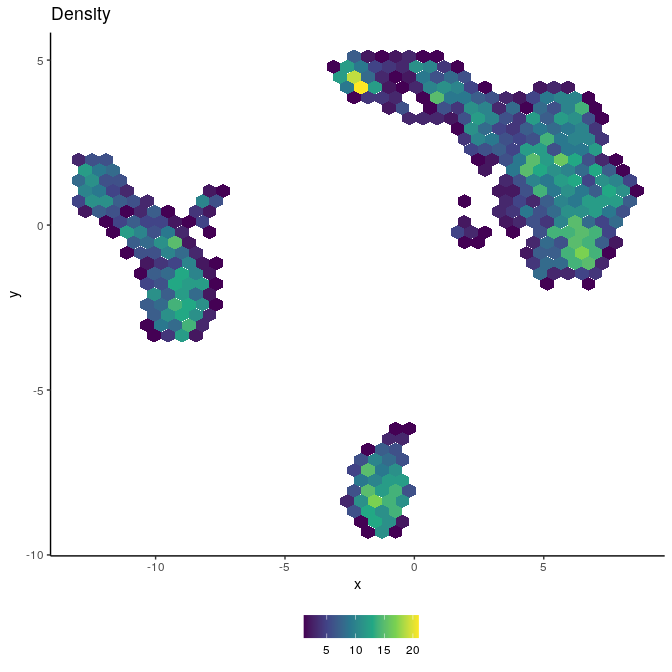#### Plot meta data in hexagon cell representation

Next I colour the hexagon cells by some meta information, such as the majority of cells cluster membership and the median total count in each hexagon cell.

plot_hexbin_meta(tenx_pbmc3k, col="cluster", action="majority")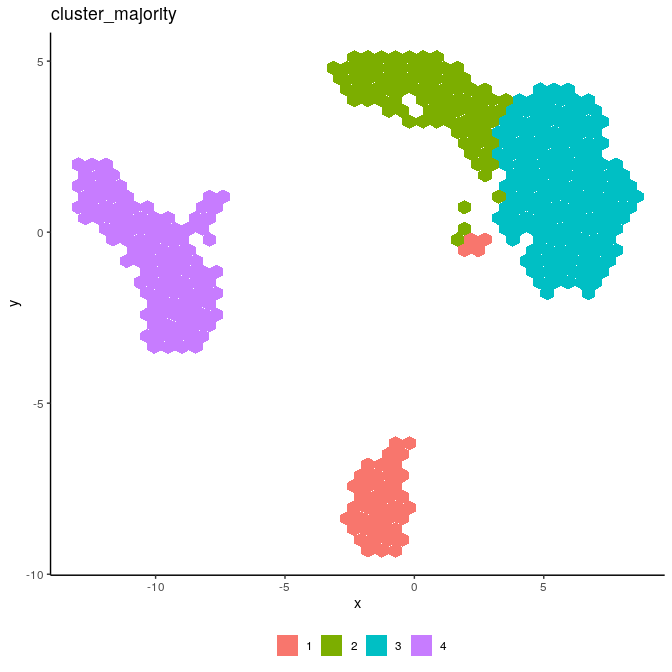plot_hexbin_meta(tenx_pbmc3k, col="total", action="median")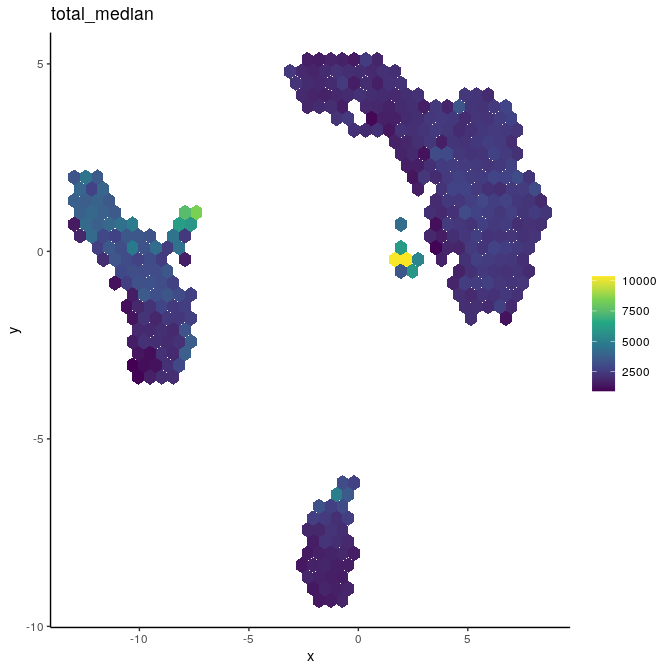While for plotting the cluster membership the outcome is not too different from the classic plot, it is much easier to observe differences in the total count.

plotUMAP(tenx_pbmc3k, colour_by="cluster")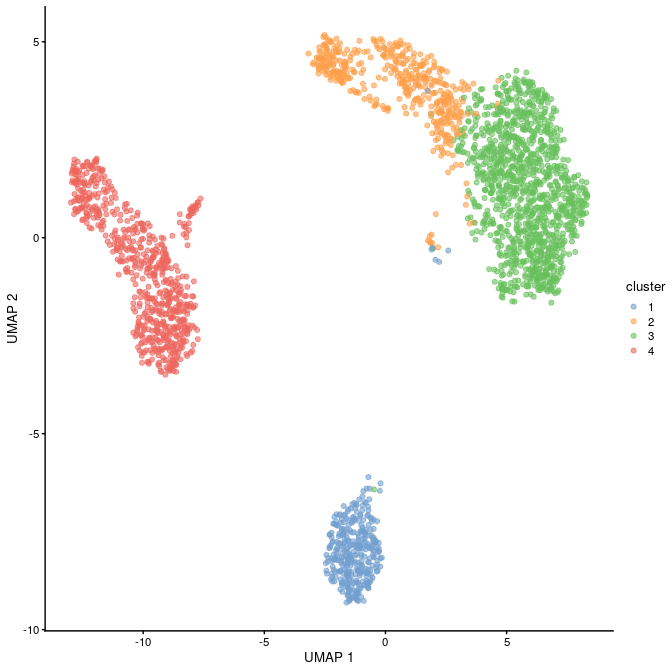plotUMAP(tenx_pbmc3k, colour_by="total")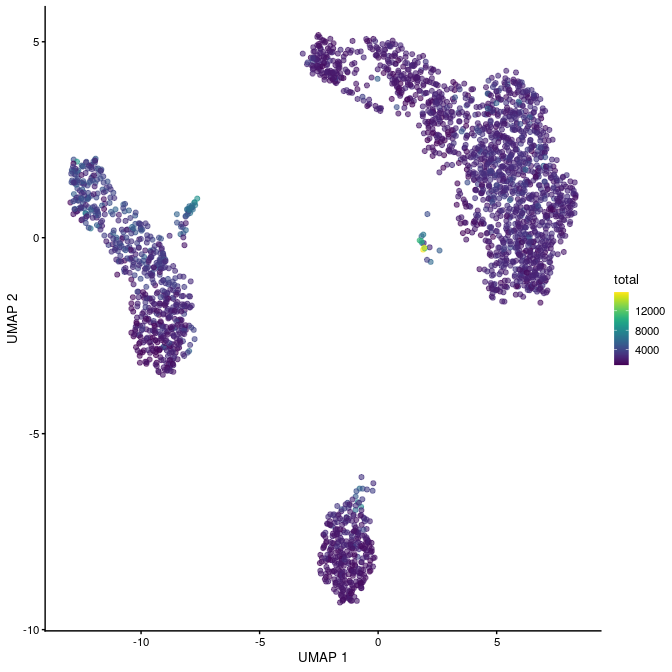For convenience there is also a function that allows the calculation of label positions for factor variables. These can be overlayed with the package ggrepel.

label_df <- make_hexbin_label(tenx_pbmc3k, col="cluster")
pp <- plot_hexbin_meta(tenx_pbmc3k, col="cluster", action="majority")
pp + ggrepel::geom_label_repel(data = label_df, aes(x=x, y=y, label = label),
colour="black",  label.size = NA, fill = NA)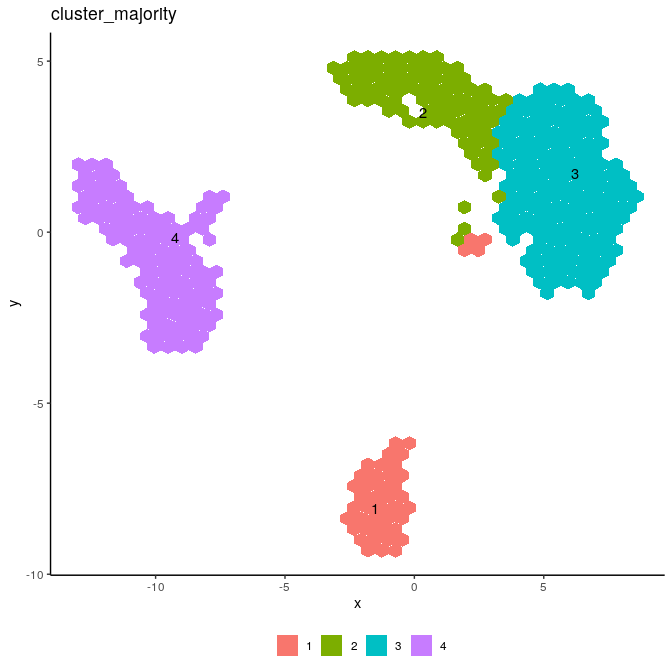#### Plot gene expression in hexagon cell representation

Finally, I will visualize the gene expression of the POMGNT1 gene in the hexagon cell representation.

gene_id <-"POMGNT1"
plot_hexbin_feature(tenx_pbmc3k, type="logcounts", feature=gene_id,
action="mean", xlab="UMAP1", ylab="UMAP2",
title=paste0("Mean of ", gene_id))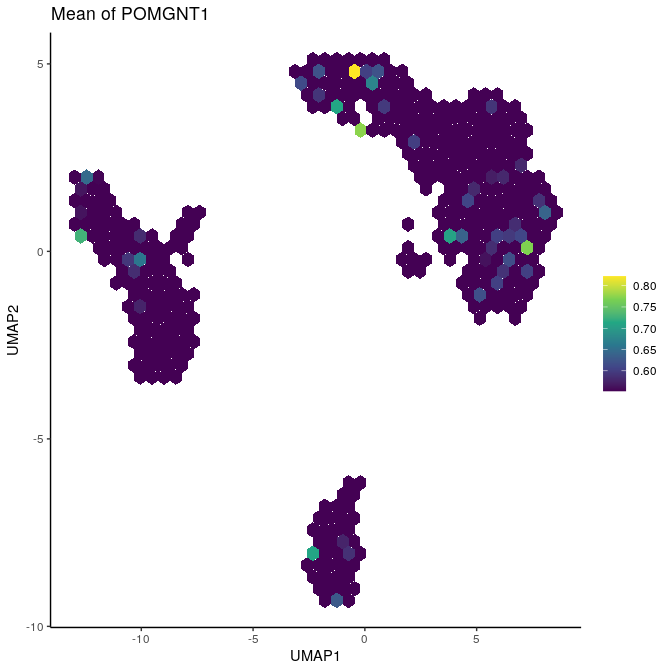Again it is much easier to observe differences in gene expression using the hexagon cell representation than the classic representation.

plotUMAP(tenx_pbmc3k, by_exprs_values="logcounts", colour_by=gene_id)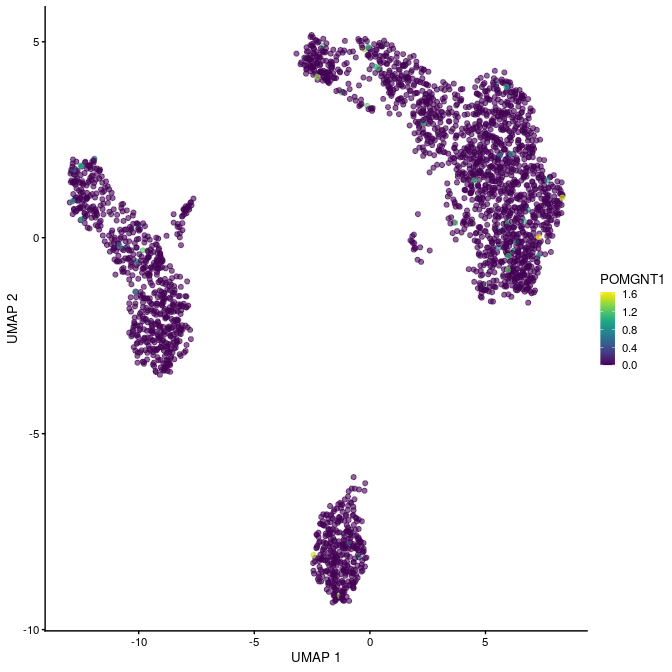We can overlay the gene expression data with the clusters for convenience.

plot_hexbin_feature_plus(tenx_pbmc3k,
col="cluster", type="logcounts",
feature="POMGNT1", action="mean")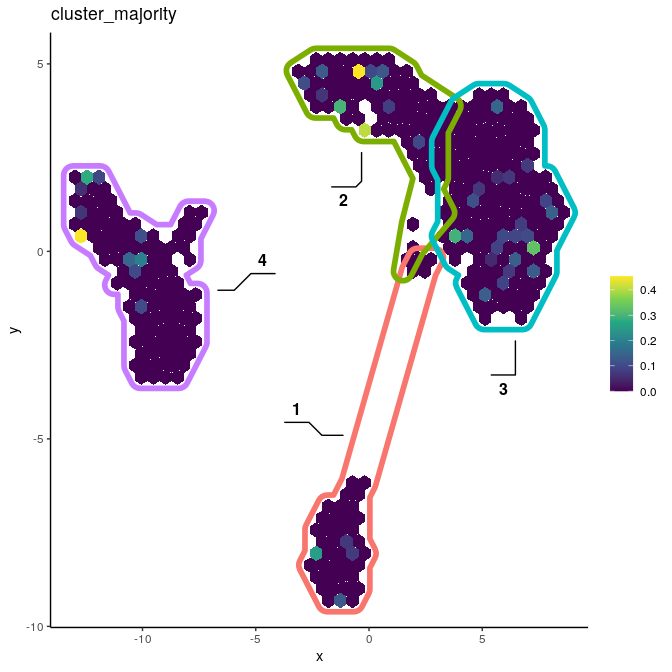### Understanding schex output as ggplot objects

The schex packages renders ordinary ggplot objects and thus these can be treated and manipulated using the ggplot grammar. For example the non-data components of the plots can be changed using the function theme.

gene_id <-"CD19"
gg <- schex::plot_hexbin_feature(tenx_pbmc3k, type="logcounts", feature=gene_id,
action="mean", xlab="UMAP1", ylab="UMAP2",
title=paste0("Mean of ", gene_id))
gg + theme_void()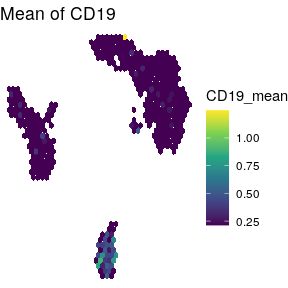The fact that schex renders ggplot objects can also be used to save these plots. Simply use ggsave in order to save any created plot.

ggsave(gg, file="schex_plot.pdf")